# Overview of package ‘bnspatial’

## Overview

This document describes the package bnspatial. The package is aimed at implementing Bayesian networks in the geographical space. Outputs are GIS ready maps of expected value (or most likely state) given known and unknown conditions specified in the network, maps of uncertainty measured as coefficient of variation or Shannon index (entropy), maps of probability associated to any states of any node in the network. bnspatial can be used any time a Bayesian network contains variables for which some sort of spatial data is available and maps are needed from the model. However, it can be useful in non-spatial context as well, for instance to discretize data before querying the network, or when many iterations and/or queries over a network are needed. Utility nodes are currently under development.
The package is designed to facilitate the spatial implementation of Bayesian networks with minimal knowledge of the R programming language. R provides a single consistent working environment, while currently available options to make maps out of Bayesian networks do not. The package acts partly as function wrapper integrating the packages raster and gRain, but offers some additional features too, including powerful parallel processing options (via foreach package) and data discretization routines. Bayesian networks must be constructed beforehand, either in R, with gRain or bnlearn packages, or via an external software, such as GeNIe (free for academic use) or Hugin, and saved in .net file format (Hugin native format); .net files can then be imported in bnspatial. Currently, .net files written with Netica are not supported, due to reading issues which are encountered by Hugin itself. A workaround is to import Netica files to GeNIe and save to .net from there. In bnspatial, Bayesian networks are objects of class grain and therefore are queried through the functions provided by the gRain package. All basic GIS tasks are performed through the raster package and spatial data are objects of its native class RasterLayer.
Bug reports and suggestions can be submitted at https://github.com/dariomasante/bnspatial/issues .

#### Acknowledgements

Development was partly funded by the European Union FP7 (2007-2013), under project ROBIN - Role Of Biodiversity In climate change mitigation (agreement 283093).

## Installation notes

bnspatial requires gRain package installed beforehand. To install, open a R session and enter the following commands:

# Use this for R version > 3.5.0
if (!requireNamespace("BiocManager")) install.packages("BiocManager")
BiocManager::install('RBGL', version = "3.8")

# ...or this for older versions:
source("http://bioconductor.org/biocLite.R")
biocLite("RBGL")

Then install bnspatial, by selecting it from the packages list, or typing in the console:

install.packages("bnspatial")

Alternatively, to install from source: - download the .tar.gz source file to the R working directory (or any other directory) - start an R session - run the following commands in the console:

## Install the required packages
# Use this for R version > 3.5.0
if (!requireNamespace("BiocManager")) install.packages("BiocManager")
BiocManager::install('RBGL', version = "3.8")

# ...or this for older versions:
source("http://bioconductor.org/biocLite.R")
biocLite("RBGL")

# Then:
install.packages("gRain", repos="http://cran.uk.r-project.org/", dependencies=T, clean=T)
install.packages("raster", repos="http://cran.uk.r-project.org/", dependencies=T, clean=T)

## Install bnspatial (full path to the file, if not in the R working directory)
install.packages("~/bnspatial_1.0.5.tar.gz", repos = NULL, type="source")

Reference manual: bnspatial.pdf
Vignettes: Overview of package ‘bnspatial’
Also from R console:

help(package=bnspatial) ## opens package index
?bnspatial ## help file for the main function

## A worked example: setting the scene

The following example shows the use of bnspatial in a hypothetical land use change context and is inspired by works like Celio et al.1. The aim is to analyse how different factors can influence land use change and where the change is most likely to occur.
A Bayesian network was built and its conditional probability table populated, in order to catch some of the relevant drivers of land use change in a sample catchment (Conwy, North Wales, UK). The links in the network reflect the relationships among variables: slope, legal status and current land use are assumed to be independent, as well as two hypothetical scenarios. One of them, intensification, predicts increase in demand and prices of agricultural products, while the other entails a more balanced management of natural resources, including measures for climate change mitigation, such as payments for carbon storage. Legal status of land determines a different level of direct or indirect involvement of stakeholders in land management and explain the link directed from it. Overall, change is defined by a combination of factors: stakeholder preferences, suitability of land for a given use (simplified with a single variable, slope) and the current land use, as a proxy for past suitability and preferences. Under protection, a given land use may or may not change, quite independently from other factors.
The simplified land use map under current and modelled conditions contains only three land use classes: arable, forest and other. The “final” land use type to be modelled depends on a set of drivers: some of them vary spatially (slope, legal status and current land use), while some other do not (socio-economic setting and stakeholder preferences). The model links the land use types to carbon stock as well, summarised under three broad categories identified as high, moderate or low.
Together with the spatial data and the network, a lookup list must be provided, to link properly the nodes from the network to the spatial data in input.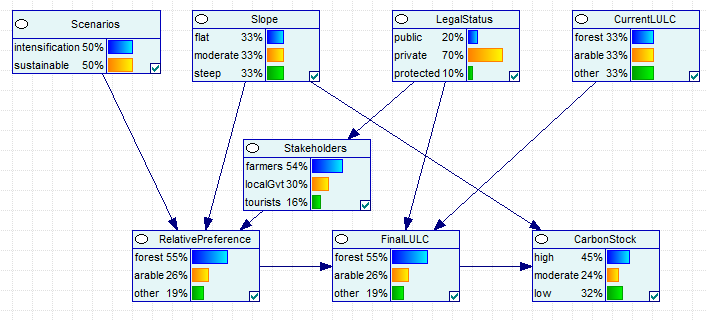The Bayesian network used in this tutorial

In this example the following questions are asked:

• What is the most likely outcome given the known conditions? What land use type can be expected under current conditions?
• If a range of conditions are set to reflect a scenario, what outcome can be expected? Are the proportions of forest and arable land expected to change under intensification or sustainable scenarios?
• What are the uncertainties associated to the modelled variable of interest (target node)? What is the likelihood of having forest and how uncertain is the land use output?
• Can we quantify the expected change in a given variable? Is carbon stock expected to increase under sustainable scenario and how is carbon stock distributed when accounting for uncertainty in the model?

Below a brief description of the data (also accessed through command ?ConwyData in R console), which are available both as raw data (i.e. file of different formats), in the extdata folder under bnspatial package directory, and as objects in native R binary format, for a quicker access and code conciseness.

• LandUseChange (LandUseChange.net): the Bayesian network.
• ConwyLU (ConwyLU.tif): a simplified version of the current land use map from the Conwy catchment (Wales, UK). It includes three classes: arable (raster value 3), forest (2), other (1).
• ConwySlope (ConwySlope.tif): a raster of slope derived from a digital elevation model at 50 meters resolution, units are degrees.
• ConwyStatus (ConwyStatus.tif): the land ownership (dummy data), divided into three possible classes: public (raster value 4), private (3), protected (1).
• evidence: a matrix, the collection of previous spatial data as extracted from each location (i.e. raster cell) in the catchment. Each value from the spatial data was discretized through dataDiscretize or bulkDiscretize functions, then assigned to the corresponding state from the Bayesian network (LandUseChange).
• LUclasses (LUclasses.txt): the look up list and classification of input spatial data (corresponding states and values). The list is formatted accordingly to bnspatial functions requirements and as returned by functions importClasses and setClasses.

In the following the main function bnspatial will be used to query the network and map the outputs. This function wraps most bnspatial functions into one, bringing directly from the network and input spatial data to the output maps. The use of bnspatial allows the user to provide external files as inputs (the Bayesian network in .net format, a look up classification file in .txt format, spatial data in various formats; see details below), and minimizes the user interaction with R and it is therefore recommended to light R users. Several additional options are available in both input and output, as shown by the tutorial below (also, type ?bnspatial in R console). Below, FinalLULC is the name of the node of interest (target), as indicated in the Bayesian network.

## Loading package and data stored in it
library(bnspatial)
data(ConwyData)
list2env(ConwyData, environment())

network <- LandUseChange
spatialData <- c(ConwyLU, ConwySlope, ConwyStatus)
lookup <- LUclasses
target <- 'FinalLULC'

## Run a spatial query on the Bayesian network
## A summary will be printed on screen to check whether spatial data correspond
## to network nodes as intended.
bn <- bnspatial(network, target, spatialData, lookup)
bn

## Plot output maps
library(raster)
par(mfrow=c(1,2))
plot(bn$Class, main='Most likely class') plot(bn$Entropy, main='Uncertainty (Shannon index)')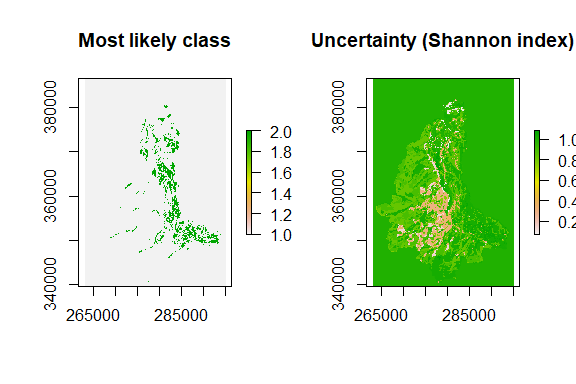## Lookup table to interpret "FinalLULC" values:
##   FinalLULC cell_ID
## 1    forest       1
## 2    arable       2
## 3     other       3

Maps from the previous example can be greatly improved by setting additional arguments, for instance msk=ConwyLU, which masks the output map to the ConwyLU only, instead of the union of all spatial inputs (default). However, the example shows how the Bayesian network is able to produce coherent outputs even under missing values, such as the case of background areas external to the catchment, for which spatial values are missing.

For ease of use, bnspatial allows the use of external files in input, which can be handy if the user resorted to external software to build the Bayesian network. In particular, the network itself and the classification file, used to link node states to the input spatial data, may be compiled more easily outside R. For conciseness, the data used in this document is loaded from the R binary format which comes with the package, i.e. was already transformed in a suitable format for direct use in bnspatial (including spatial data). However, the same data are provided in raw format as well (see sub-directory extdata under bnspatial directory).
In fact, a more common case is when spatial data in typical formats are available to the user (e.g. .tif), Bayesian network was built using a stand alone software (e.g. GeNIe, Hugin or Netica) and structure, states and CPT were defined within that. bnspatial allows to use such inputs; if the example above resorted to external data, it would be as follows, with identical outputs:

## Loading data stored in package
network <- 'fullPath/toPackageLibrary/bnspatial/extdata/LandUseChange.net'

spatialData <- c('fullPath/toPackageLibrary/bnspatial/extdata/CurrentLULC.tif',
'fullPath/toPackageLibrary/bnspatial/extdata/degSlope.tif',
'fullPath/toPackageLibrary/bnspatial/extdata/LegalStatus.tif')

lookup <- 'fullPath/toPackageLibrary/bnspatial/extdata/LUclasses.txt'

## Run a spatial query on the Bayesian network
bn <- bnspatial(network, 'FinalLULC', spatialData, lookup)

'fullPath/toPackageLibrary/' stands for the full path to the package library sub-directory, under R directory.

The text file (lookup argument) is the only object that the user has to build from scratch. This can be done with any text editor, then imported for use in bnspatial with fucntion importClasses. Alternatively, the user can build it directly in R by using the function setClasses, then optionally export to .txt for future reference setting argument wr.

As a general guideline, the text file should be formatted as follows, for each node:

First line: the node name.
Second line: the node states, comma separated (spaces allowed).
Third line: interval values from the spatial data associated to the states (integer values for discrete data; interval boundaries, including endpoints, for continuous data). The same exact order as node states is required.

In the above example, the external .txt appears as follows:
CurrentLULC
forest,other,arable
2, 1, 3
Slope
flat, moderate, steep
-Inf, 1, 7, Inf
LegalStatus
public, private, protected
4, 3, 1

Please refer to help pages for setClasses and importClasses for further details (?setClasses from R console).

Under current release, Bayesian networks from external software must be provided in .net format. The use of either GeNIe (free for academic use) or Hugin is recommended to build the Bayesian networks, as both can export them successfully to the .net format (.net is the native format for Hugin). Currently, .net files written by Netica 5.24 are not supported, due to loading issues which are encountered by Hugin itself. A workaround is to open Netica networks with GeNIe and export to .net from there.
The use of gRain or bnlearn packages is recommended for building and manipulating Bayesian networks in the R environment. If the network was built with bnlearn, through the function as.grain it can be exported to an object of class grain, suitable for bnspatial.
All input spatial data must be in raster format, no vector data is accepted. Categorical data in shapefile format, for instance, must be converted to raster. Functionalities to accept other file formats for networks and vector data formats are planned for future releases. Packages rgdal, raster and sp offer a great deal of GIS tools for R, including a complete range of read/write functionalities.

## Output maps

By default, outputs of bnspatial are the expected state of target node (i.e. the state with the highest relative probability) and the uncertainty, expressed as entropy by the Shannon index.
Other outputs can be obtained, by adding or removing the following to the argument what:

• class (default) returns the relatively most likely state of the target node (expected state).
• entropy (default) calculates the Shannon index and returns the entropy given the state probabilities: $-\sum_{i=1}^n p_i ln(p_i)$ where $$p_i$$ is the probability associated to the state $$i$$ of target node.
• probability returns a map of probability for each (selected) state of the target node.
• expected returns the expected value for the target node. Only valid for continuous target nodes. midValues argument must be provided. The expected value is calculated by summing the mid values of target node states weighted by their probability: $p_1 * mid_1 + p_2 * mid_2 + ... + p_n * mid_n$ where $$mid_n$$ is the mid value of the $$n^{t_h}$$ state of a node.
• variation returns the coefficient of variation, as a measure of uncertainty: $\frac{\sqrt{\sum_{i=1}^n (mid_i - val)^{2} * p_i}}{val}$ where $$val$$ is the expected value as calculated by what='expected' (above).

So for instance, the following command will produce two maps, one of probability of land use to be forest and the other for arable:

bn <- bnspatial(network, 'FinalLULC', spatialData, lookup, msk=ConwyLU,
what="probability", targetState=c("arable","forest"))
par(mfrow=c(1,2))
plot(bn$Probability$forest, main="Probability of forest")
plot(bn$Probability$arable, main="Probability of arable land")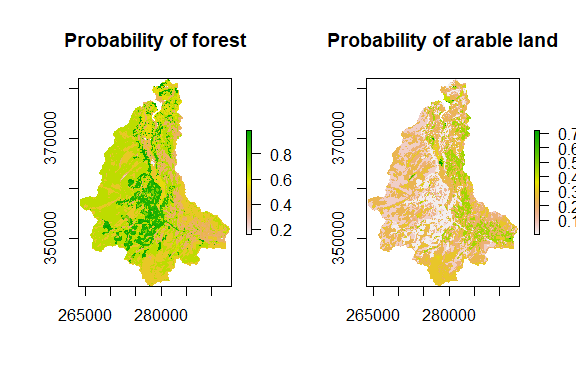Note that if targetState argument was not specified (default is NULL), maps of probability for all states of the target node would be returned.

## Exploring scenarios: setting node evidence

The user may be interested in exploring the likelihood of outcome for a target node, given certain conditions. To do so, any node can be fixed to a given state and maps can be produced accordingly, by setting them as additional arguments. So, drawing from the main example, we may be interested in mapping the most likely land use of preference by farmers, under the intensification scenario and compare that with the sustainable scenario. To do so it is sufficient to set target="RelativePreference" and the two additional arguments Stakeholders="farmers" and Scenarios="intensification" (then "sustainable" for the comparison)

par(mfrow=c(2,2))

bn <- bnspatial(network, "RelativePreference", spatialData, lookup, msk=ConwyLU,
Scenarios="intensification")
plot(bn$Class, main="Intensification scenario") plot(bn$Entropy, main="Uncertainty (Shannon index)")

bn <- bnspatial(network, 'RelativePreference', spatialData, lookup, msk=ConwyLU,
Scenarios="sustainable")
plot(bn$Class, main="Sustainability scenario") plot(bn$Entropy, main="Uncertainty (Shannon index)")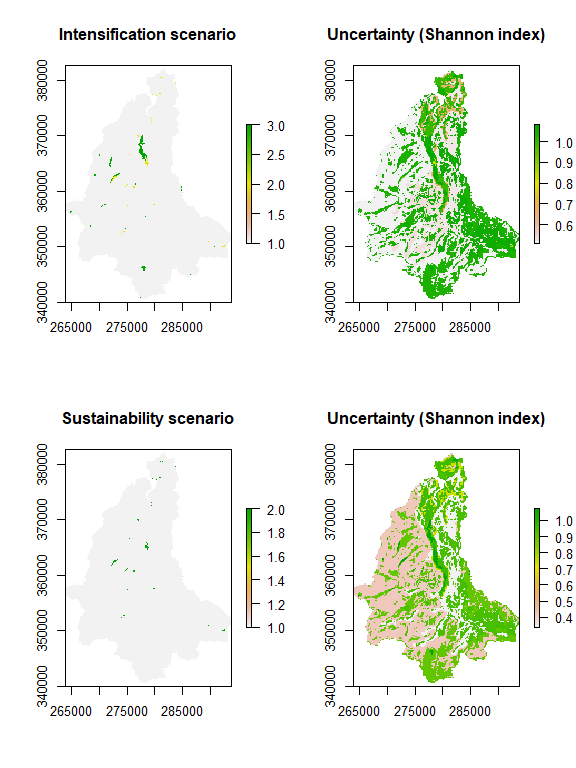No big differences are noticed, mainly because preferences do not change sensibly in relative terms. However uncertainty of outcome is quite different between the two scenarios. This is further explained by mapping the probabilities of preference for forest and arable under the two scenarios:

bn <- bnspatial(network, "RelativePreference", spatialData, lookup, msk=ConwyLU,
what="probability", targetState=c("forest","arable"), Scenarios="intensification")
plot(bn$Probability$forest, main="P of forest preference (intensif.)")
plot(bn$Probability$arable, main="P of arable preference (intensif.)")

bn <- bnspatial(network, 'RelativePreference', spatialData, lookup, msk=ConwyLU,
what="probability", targetState=c("forest","arable"), Scenarios="sustainable")
plot(bn$Probability$forest, main="P of forest preference (sustain.)")
plot(bn$Probability$arable, main="P of arable preference (sustain.)")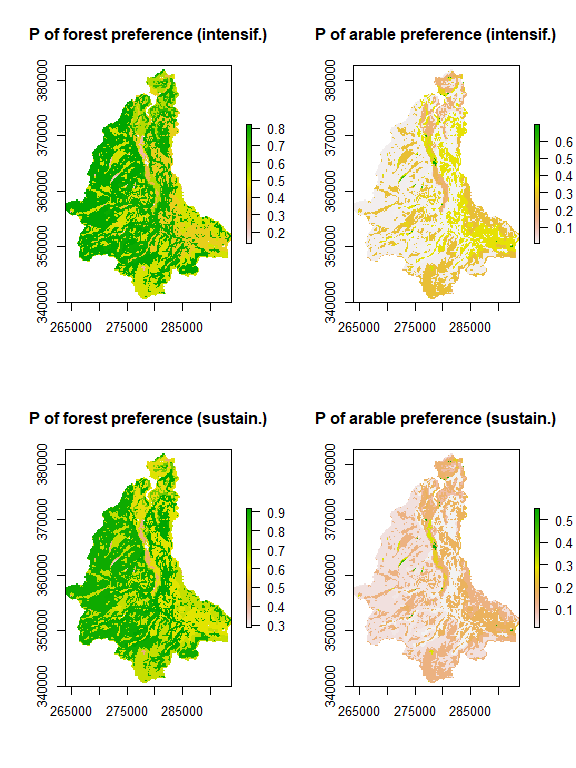Now a further question can be asked, in particular how carbon stock is expected to be affected by land use under different scenarios and how much carbon we can expect under different scenarios. To answer the question and have quantitative maps, additional information about the meaning of the carbon stock classes is needed. More specifically, a value of carbon stock must be assigned to each of the three classes:

• high carbon stock is considered high when above 120 tons per hectare. A maximum is set at 230 t/ha, as values above that are quite unlikely. The value of reference for this class is therefore in between the outer values: 175 tons per hectare
• moderate when between 30 and 120 t/ha, therefore a mid value of 75 t/ha is the reference for this class
• low when ranging between 0 to 30 t/ha, setting a class mid value at 15 t/ha for this class.
midValues <- c(175, 75, 15)

bn <- bnspatial(network, "CarbonStock", spatialData, lookup, msk=ConwyLU,
midvals=midValues, what=c("expected","variation"), Scenarios="intensification")
plot(bn$ExpectedValue, main="Expected carbon (t/ha) (intensif.)") plot(bn$CoeffVariation, main="Uncertainty (coeff. variation)")

bn <- bnspatial(network, "CarbonStock", spatialData, lookup, msk=ConwyLU,
midvals=midValues, what=c("expected","variation"), Scenarios="sustainable")
plot(bn$ExpectedValue, main="Expected carbon (t/ha) (sustain.)") plot(bn$CoeffVariation, main="Uncertainty (coeff. variation)")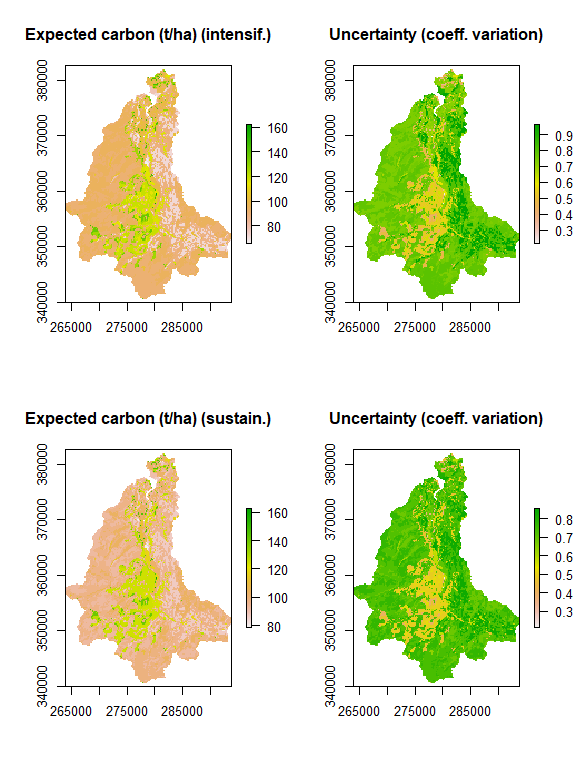It should be noted that this model does not return a quantitative estimate of carbon as it may be in a deterministic or process based model, but rather a quantitative expectation of carbon stock. That is the estimate based on uncertainty. For instance, a cell in the output raster may have any value between 15 and 175, but its land use class would be only one of three (forest, arable or other). However, the probabilities of having any of the three land use classes would vary and this variation is accounted for in the carbon stock estimate. This is not a generally valid statement and the meaning of the expected value of a target node depends on the model structure and variables.

In the examples above the function bnspatial was used exclusively, but it can be quite inefficient when multiple options have to be tested, or the spatial data involved is big enough to require significant computing time. An option is to resort to parallel processing, by setting argument inparallel=TRUE (see next paragraph), or making sure that the query is run on the area of interest only, by setting argument msk as already shown.
However, these options do not prevent bnspatial from repeatedly loading and discretize the same data over and over, which can be a very time consuming process. To avoid that, using directly the wider set of functions provided by bnspatial is recommended, instead of their wrapper bnspatial.

## Speeding up: parallel processing

When the scale of analysis is very wide or spatial data has high resolution, computation time can be significant. To speed up the network querying, parallel processing options are provided in bnspatial, integrating the powerful functions from foreach package and applying them in complete autonomy. When using parallel processing options, care should be paid to the RAM commitment, as memory should be sufficient to contain all data being processed in parallel (use of memory can be easily checked from Task Manager in Windows systems). Solutions to this issue are currently under development.
To apply parallel processing, simply set inparallel=TRUE. By default the number of tasks will be the number of cores/processors available minus one. If a different number of tasks is required, that number can be set, e.g. inparallel=2 will use two cores. A higher number of tasks than number of cores/processors can be provided, but there are potential shortcomings and the user should avoid that choice, unless knowing exactly the reason for it.

bn <- bnspatial(network, 'FinalLULC', spatialData, lookup, inparallel=2)

## Exporting spatial data

All the spatial data created with bnspatial can be easily exported to a portable format, suitable for use in any GIS. The task of writing data are handled entirely by rgdal and raster packages. Available file formats are listed for function writeFormats, from raster package.
In bnspatial, data can be exported indirectly, by creating the output data and then exporting via writeRaster function available from raster package, or directly with bnspatial and mapTarget functions, which will write the data to the directory and format of choice. For both functions, this is done by setting arguments exportRaster and path: when exportRaster=TRUE, rasters will be exported to default format .tif and stored at the working directory. A character can be provided instead of the logical value, specifying another preferred extension (e.g. ".asc"), in which case the raster will be exported to that format.
If argument path is not specified, output files will be written to the working directory as per getwd().
File names can’t be provided, as they are set automatically, depending on the output required by what and accordingly to the following naming convention:

• "class" will set the exported file name to <target node name>_Class.<file format>
• "entropy" to <target node name>_ShanEntropy.<file format>
• "probability" to <target node name>_Probability_<target state>.<file format>
• "expected" to <target node name>_ExpectedValue.<file format>
• "variation" to <target node name>_CoefVariation.<file format>

An additional comma separated file (.csv) is written to the same directory when what argument is "class", providing a key to relate the raster values to the node states they refer to.

## Unfolding bnspatial functions

The function bnspatial is actually a wrapper for a bunch of other functions. Below the same example as above is used, but using explicitly the functions underneath bnspatial. These can all be accessed independently from the user and offer many more options to handle and process the network spatially.

### loadNetwork

The first step is loading the Bayesian network from an external file format. In the example, LandUseChange.net can be found at the extdata subfolder of bnspatial, under the R package library directory. To load the network:

network <- loadNetwork(LandUseChange, target='FinalLULC')
network

An object of class grain is returned. Optional argument target should be set when known, as it will speed up the network query remarkably.

### setClasses and importClasses

These functions are meant to import, read or export a list or a text file, containing the known nodes and the values delimiting their respective states. Outputs returned by this functions are exactly the same, to be used for the integration and error checking of Bayesian network and input spatial variables. See ?setClasses for details. Here we use a simple txt file and import it:

fullpath <- system.file("extdata", "LUclasses.txt", package = "bnspatial")
fullpath

intervals <- importClasses(fullpath)
intervals

### linkNode and linkMultiple

linkNode links a node of the Bayesian network to its corresponding spatial dataset (in raster format), returning a list of objects, including the spatial data and relevant information about the node. linkMultiple operates over multiple rasters and nodes, and requires a look up list to match correctly spatial data and related knodes of the network (this list is easily generated with setClasses or importClasses).

spatialData <- c(ConwyLU, ConwySlope, ConwyStatus)
spatialDataList <- linkMultiple(spatialData, network, intervals)

### aoi

Create a mask within which the query is performed. Not all cells of a geographical area may be of interest and just passing them all to the query and mapping functions may significantly increase processing time and return unwanted coverages. This function creates a raster (or coordinates of points) defining the area of interest using a user defined mask, whose resolution and extent will be inherited to the output maps. When msk is not specified input rasters will be united to the finest resolution among them and to the maximum extent enclosing them. This may potentially create huge rasters, so it is better to provide a mask. A subset of the data can be provided with argument mskSub, if a subset of values from a layer has to be used as mask. Below we use one of the input rasters as mask, defining outline, extent and resolution of final maps. Note also argument xy, which will be used to return coordinates of valid cells from mask, rather than a raster, as the mask is just an intermediate step and no spatial data is required. This way will speed up processing and avoid the creation of unnecessary objects in memory.

## Return coordinates of valid cells inside the mask instead of a raster layer
msk <- aoi(spatialData, msk=ConwyLU, xy=TRUE)
head(msk)

### extractByMask

This function extracts the values from a given input raster based on a mask. Can be useful for data analysis on rasters and generic GIS tasks.

m <- aoi(msk=ConwyLU, mskSub=c(2,3))
head( extractByMask(ConwySlope, msk=m), 20)

### dataDiscretize ; bulkDiscretize

These functions discretize continuous input data into classes. Classes can be defined by the user or, if the user provides the number of expected classes, calculated from quantiles (default option) or by equal intervals.

s <- runif(30)

## Split by user defined values. Values out of boundaries are set to NA:
dataDiscretize(s, classBoundaries = c(0.2, 0.5, 0.8))

### queryNet ; queryNetParallel

Queries the Bayesian network and returns the probabilities for each state of the target node. Available input variables are set as evidence. queryNetParallel works as queryNet, but makes use of multi cores/processors facilities for big network queries, by splitting data into chunks and processing them in parallel. Static evidence can be provided for any node of the network independently from availability of spatial data. This is useful to test scenarios for instance, where the user may control specific nodes (type ?queryNet in the console to see an example).

q <- queryNet(network, 'FinalLULC', evidence)
head(q)

### mapTarget

This function creates the required spatial outputs for the target node. By default most relatively likely class and entropy (uncertainty) associated are returned. More options available (see ?mapTarget), including returning matrix of coordinates and values rather than spatial data (spatial=TRUE) or automatic export to any of the common GIS raster format (exportRaster=TRUE will save rasters as .tif by default).

target <- 'FinalLULC'
statesProb <- queryNet(network, target, evidence)
maps <- mapTarget(target, statesProb, msk=ConwyLU)
maps

par(mfrow=c(1,2))
plot(maps$Class, main='Most likely land use class') plot(maps$Entropy, main='Uncertainty (Shannon index)')

1. Celio, E., Koellner, T., & Gret-Regamey, A., 2014. Modelling land use decisions with Bayesian networks: Spatially explicit analysis of driving forces on land use change. Environmental Modelling & Software, 52, 222-233.